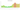# د.ت.‏

## Tunisian Dinar

Tunisia's unit of currency is the dinar. There are 1000 milim or millimes within it. Given that French is widely spoken in Tunisia and that DT is derived from French, the acronym DT is regularly used there, however putting "dinar" after the amount is also acceptable. The abbreviation TD is also used occasionally but is less commonly used.

#### how has the value of the currency changed in the last year?## USD 1 = TND 3.167

How much is10 US dollars worth inTunisian dinars?
At the current exchange rate, 10 US dollars is worth 31.67 Tunisian dinars
How much is50 US dollars worth inTunisian dinars?
At the current exchange rate, 50 US dollars is worth 158.35 Tunisian dinars
How much is100 US dollars worth inTunisian dinars?
At the current exchange rate, 100 US dollars is worth 316.7 Tunisian dinars
How much is500 US dollars worth inTunisian dinars?
At the current exchange rate, 500 US dollars is worth 1,583.5 Tunisian dinars
How much is2,000 US dollars worth inTunisian dinars?
At the current exchange rate, 2,000 US dollars is worth 6,334 Tunisian dinars
How much is10 US dollars worth inTunisian dinars?
At the current exchange rate, 10 US dollars is worth 31.67 Tunisian dinars
How much is50 US dollars worth inTunisian dinars?
At the current exchange rate, 50 US dollars is worth 158.35 Tunisian dinars
How much is100 US dollars worth inTunisian dinars?
At the current exchange rate, 100 US dollars is worth 316.7 Tunisian dinars
How much is500 US dollars worth inTunisian dinars?
At the current exchange rate, 500 US dollars is worth 1,583.5 Tunisian dinars
How much is2,000 US dollars worth inTunisian dinars?
At the current exchange rate, 2,000 US dollars is worth 6,334 Tunisian dinars